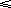#### You may also like### Doodles

Draw a 'doodle' - a closed intersecting curve drawn without taking pencil from paper. What can you prove about the intersections?### Russian Cubes

I want some cubes painted with three blue faces and three red faces. How many different cubes can be painted like that?### Picture Story

Can you see how this picture illustrates the formula for the sum of the first six cube numbers?

# Ordered Sums

##### Age 14 to 16Challenge Level

Let a(n) be the number of ways of expressing the integer n as an ordered sum of 1's and 2's. For example, a(4) = 5 because:

 4 = 2 + 2 2 + 1 + 1 1 + 2 + 1 1 + 1 + 2 1 + 1 + 1 + 1.

Let b(n) be the number of ways of expressing n as an ordered sum of integers greater than 1.

 (i) Calculate a(n) and b(n) for n8. What do you notice about these sequences? (ii) Find a relation between a(p) and b(q). (iii) Prove your conjectures.